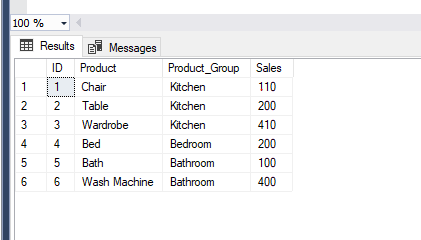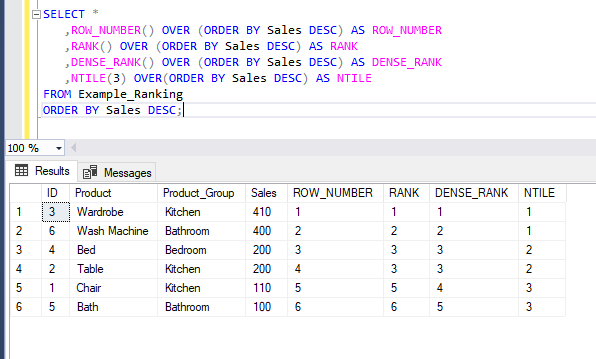• 17. 11. 2019
• Ing. Jan Zedníček - Data & Finance
• 0

SQL ranking functions enable us to rank records in the table based on values of a field or multiple fields. Clause OVER() is mandatory for the ranking functions. Ranking functions belongs to the group of Window functions. Aggregate functions and Offset functions (for paging) also belong to this group.

## List of Ranking Functions

• ROW_NUMBER () OVER (PARTITION BY | ORDER BY) – returns serial number of the row with possibility of division into parts (partitions) and sorting. It starts from 1 for every group.
• RANK () OVER (PARTITION BY | ORDER BY) – returns the rank of every record part by part (partitions)
• DENSE_RANK () OVER (PARTITION BY | ORDER BY) – returns the rank of every record part by part (partitions) without gaps between ranks
• NTILE () OVER (PARTITION BY | ORDER BY) – divides rows into n groups depending on the argument

## How to Use Ranking Functions

To use a ranking function, you have to follow these steps:

1. Apply one of the functions ROW_NUMBER, RANK, DENSE_RANK, NTILE
2. Attach clause OVER (ORDER BY). By doing that, we define over which field will we count the rank in ascending or descending order (mandatory)
3. We can add PARTITION BY into the OVER() clause. Doing this will create groups. Rank will then be generated for each group separately (optional)

The practical application of ranking functions can be shown best on an example. We will create testing table with sales and products and fill it with data.

`USE tempdb;`
`CREATE TABLE Example_Ranking`
`(ID INT IDENTITY(1,1),`
`Product VARCHAR(100),`
`Product_Group VARCHAR(100),`
`Sales NUMERIC);`

`INSERT INTO Example_Ranking (Product, Product_Group, Sales)`
`VALUES ('Chair', 'Kitchen', 110),`
`('Table', 'Kitchen', 200),`
`('Wardrobe', 'Kitchen', 410),`
`('Bed', 'Bedroom', 200),`
`('Bath', 'Bathroom', 100),`
`('Wash Machine', 'Bathroom', 400);`We will then apply functions on this table. We will then add field with functions into the established table. We will not be applying PARTITION BY clause to make things easier.

`SELECT *`
`   ,ROW_NUMBER() OVER (ORDER BY Sales DESC) AS ROW_NUMBER`
`   ,RANK() OVER (ORDER BY Sales DESC) AS RANK`
`   ,DENSE_RANK() OVER (ORDER BY Sales DESC) AS DENSE_RANK`
`   ,NTILE(3) OVER (ORDER BY Sales DESC) AS NTILE`
`FROM Example_Ranking`
`ORDER BY Sales DESC;`Interpretation

• ROW_NUMBER() – no item has the same rank and there are no gaps between them
• RANK() – items can have the same rank and there are gaps between ranks
• DENSE_RANK() – items can have the same rank and there are no gaps between ranks
• NTILE(3) – divides records into 3 numerically identical groups